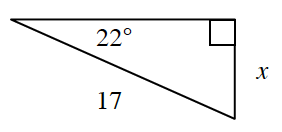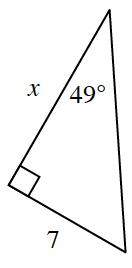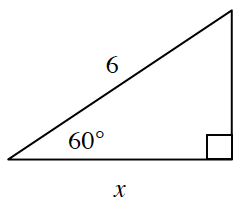### Home > GC > Chapter 5 > Lesson 5.1.2 > Problem5-16

5-16.

For each triangle below, write an equation relating the reference angle (the given acute angle) with the two side lengths of the right triangle. Then solve your equation for $x$.

Refer to the Math Notes box in Lesson 5.1.2 if you need help writing an equation relating to a reference angle.
It will be helpful for all three parts of this problem.

1.$\sin\theta=\frac{\text{opp}}{\text{hyp}}$

$\text{sin}(22^{\circ}) = \frac{\it{x}}{17}$

$17\text{ sin}(22^\circ) = \it{x}$

$x \approx 6.37$

1.$\text{tan}\theta = \frac{\text{opp}}{\text{adj}}$

$\text{tan}(49º) = \frac{7}{\textit{x}}$

$x\text{ tan}(49º) = 7$

$\frac{x\tan(49º)}{\tan(49º)}=\frac{7}{\tan(49º)}$

$x = \frac{7}{\text{tan}(49^\circ)}$

$x \approx 6.09$

1.Follow the same steps as in parts (a) and (b).